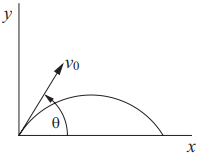## Projectile Motion

Consider a spectator at a hockey game hurls a projectile towards the ice rink at an angle of 67° to the horizontal. If the object leaves the spectator’s hand at 5 m/s, what is the horizontal velocity?

HintHint 2
$$v_x=v_0cos(\theta )$$$where $$v_x$$ is the horizontal velocity, $$v_0$$ is the initial velocity, and $$\theta$$ is the angle. Because the object is thrown, the projectile has constant acceleration. Thus, $$v_x=v_0cos(\theta )$$$
where $$v_x$$ is the horizontal velocity, $$v_0$$ is the initial velocity, and $$\theta$$ is the angle.$$v_x=(5\frac{m}{s})cos(67^{\circ})=(5\frac{m}{s})(0.391)=1.95\:m/s$$\$
1.95 m/s